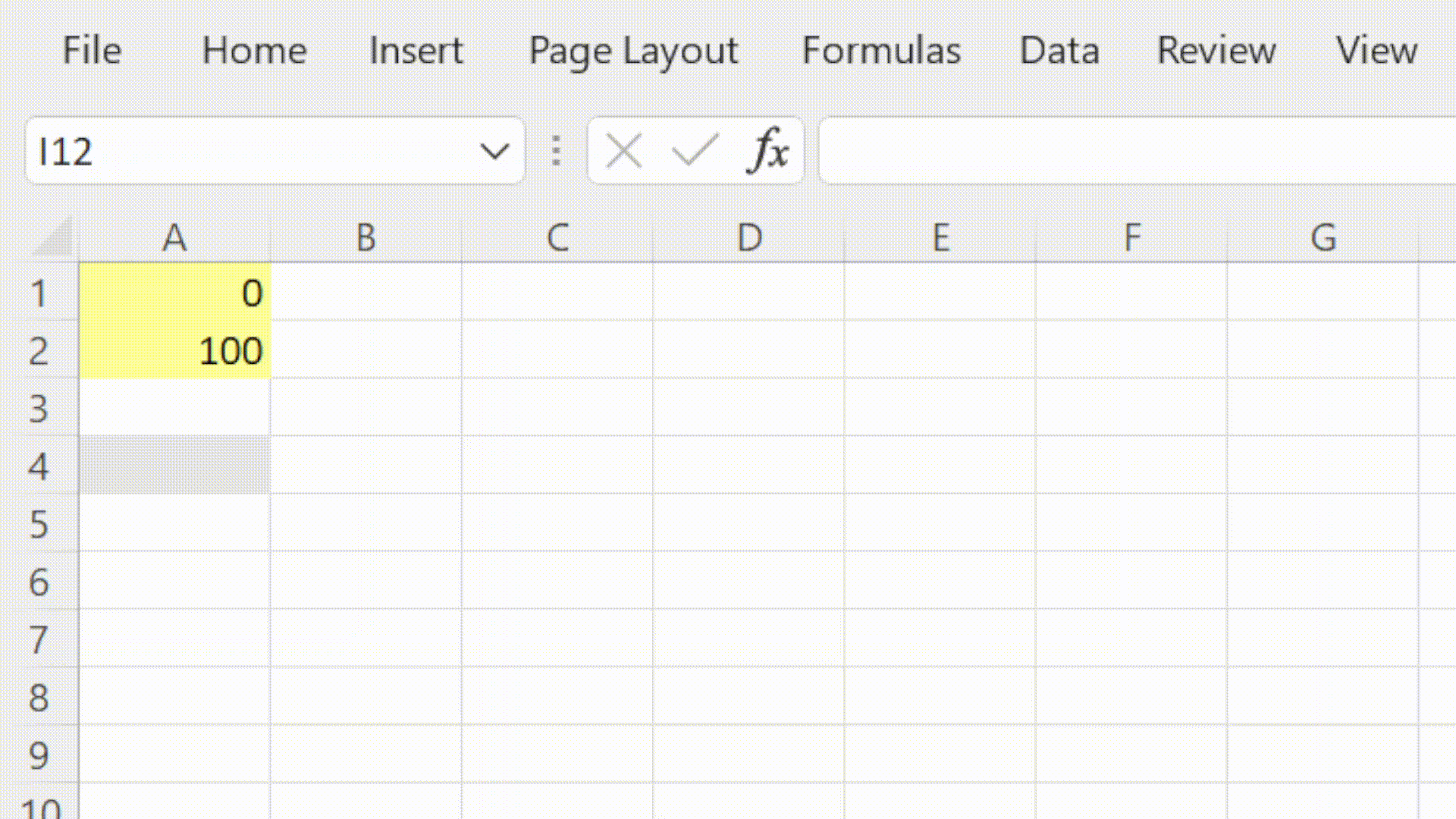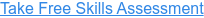Back to Blog# How to use Excel's MAX Function

Published
Jun 11, 2021 10:52:00 AM

Returns the largest value from a range of numbers

#### What does Excel's MAX function do?

The MAX Function is one of Excel's Statistical functions.

The MAX function returns the largest value in the range of numbers provided.

In financial modelling the MAX function is very useful for ensuring that the result of a calculation does not go below a given threshold. It is often used to ensure that a balance does not go below zero for example.

#### Here's a simple example

Let's take a look at a simple example of the MAX function.

We would like to know the largest number in cells A1 and A2 (or that range A1 to A2).

##### =MAX(A1, A2)  or  =MAX(A1:A2)#### What does that mean in plain English?

Return the largest number from the values stored in cells A1 and A2 (or in the range A1 to A2).

#### What to consider when using the MAX function in your financial model

• Values can be hardcoded, selected singularly (up to 255) or as ranges.
• MAX ignores empty cells, text values and TRUE & FALSE.
• Use the MAXA function if you would like to include empty cells, text values and TRUE & FALSE

Read more about the MAX function on the Microsoft support page here.

#### Take our free 30-question skills assessment

See where you stand with your Excel, financial modelling, data visualisation & analytics, and modelling tech skills